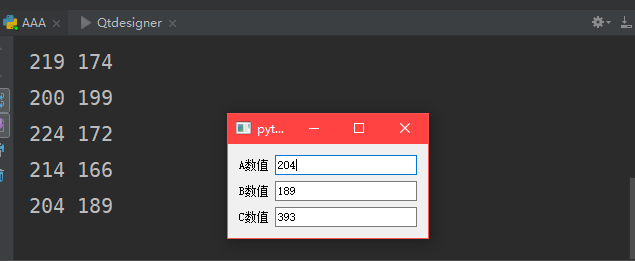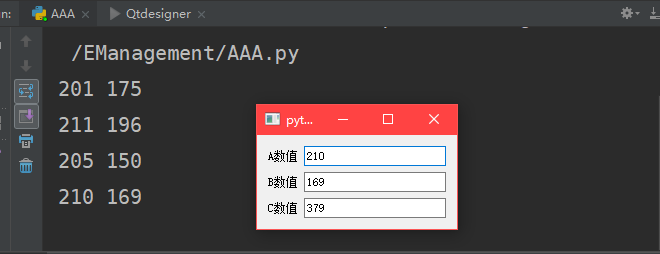# 基于Python3和pyqt5实现控件数据动态显示

import sys
import random
import numpy as np
from time import sleep
import datetime
from PyQt5 import QtWidgets
from PyQt5.QtCore import *
from PyQt5.QtGui import *
from PyQt5.QtWidgets import *


# 定义数据生成线程，该线程主要目的是不断生成随机数，用来模拟不断采集数据

class WorkThread(QThread):
# 初始化线程
def __int__(self):
#线程运行函数
def run(self):
while True:
global T_value
global P_value
T_value = random.randint(200,225)
P_value = random.randint(150,200)
print(T_value, P_value)
sleep(3)


# 定义显示面板

class plotwindows(QtWidgets.QWidget):
def __init__(self):
super(plotwindows,self).__init__()
layout = QFormLayout()
self.edita3 = QLineEdit()
self.edita4 = QLineEdit()
self.edita5 = QLineEdit()
self.setLayout(layout)
self.Mytimer()


# 定义定时器，使用定时器每隔一段时间来出发update函数，从而刷新数据

def Mytimer(self):
timer = QTimer(self)
timer.timeout.connect(self.update)
timer.start(100)


# 刷新函数

def update(self):
self.edita3.setText(str(T_value))
self.edita4.setText(str(P_value))
global SUM_value
SUM_value = T_value + P_value
self.edita5.setText(str(SUM_value))

def mainwindows():
app =QtWidgets.QApplication(sys.argv)
new = plotwindows()
new.show()
sys.exit(app.exec_())

if __name__ == "__main__":